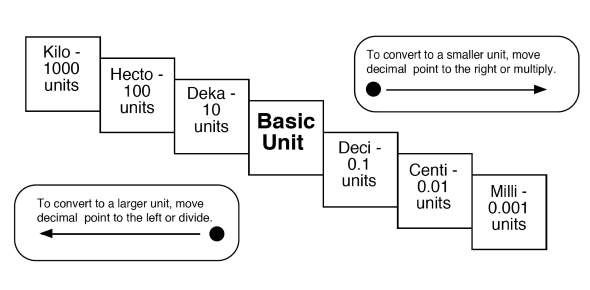# Metric System And Conversion Quiz!

10 Questions | Total Attempts: 241SettingsThe metric system is an alternative system of measurement used in most countries. The metric system uses three basic units from which all others are calculated, and these are grams for weight, meters for length and seconds for time. Take up the test below and see what you understand on the metric system and metric conversions with some examples. All the best!

• 1.
True or False: The metric volume of a liquid is measured in Liters.
• A.

True

• B.

False

• 2.
It would make sense to measure your desk using :
• A.

Kilometers

• B.

Millimeters

• C.

Centimeters

• D.

Meters

• 3.
Use the King Henry slide or ladder method to solve: 1000 mg = _____________ g
• A.

.01 g

• B.

1 g

• C.

100 g

• D.

10 g

• 4.
The metric system is based on units of powers of __________
• 5.
Use the  King  Henry slide or ladder method to solve: 180 cm = _________________ mm
• A.

180 mm

• B.

18 mm

• C.

.180 mm

• D.

1,800 mm

• 6.
Use the  King  Henry slide or ladder method to solve: 209 g = ___________ kg
• A.

.209 kg

• B.

2,090 kg

• C.

209,000 kg

• D.

2.09 kg

• 7.
Use the  King  Henry slide or ladder method to solve: 15 km = ____ m
• A.

.15 m

• B.

1,500 m

• C.

.015 m

• D.

15,000 m

• 8.
Use the  King  Henry slide or ladder method to solve: 8.3 m = _________  cm
• A.

.830 cm

• B.

830 cm

• C.

8,300 cm

• D.

83 cm

• 9.
Use the King Henry slide or ladder method to solve: 1200 mL = _____________ L
• A.

0.12 L

• B.

1.2 L

• C.

12 L

• D.

120 L

Related TopicsBack to top